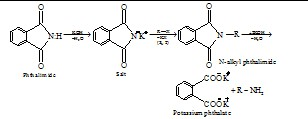# The increasing order of nitration of the following compounds is :Option 1) (b) < (a) <  (c) < (d) Option 2) (a) < (b) <  (c) < (d) Option 3) (b) < (a) < (d) < (c)  Option 4) (a) < (b) < (d) < (c)

V Vakul

As we learnt in

Gabriel Phthalimide Synthesis -

Further alkylation can be stopped and a pure primary amine can be obtained by alkylation of phthalimide ( Gabriel synthesis ) followed by hydrolysis.

- whereinHere the aniline is least reactive due to formation of anilinium ion in acidic medium.Option 1)

(b) < (a) <  (c) < (d)

Option 2)

(a) < (b) <  (c) < (d)

Option 3)

(b) < (a) < (d) < (c)

Option 4)

(a) < (b) < (d) < (c)

Exams
Articles
Questions